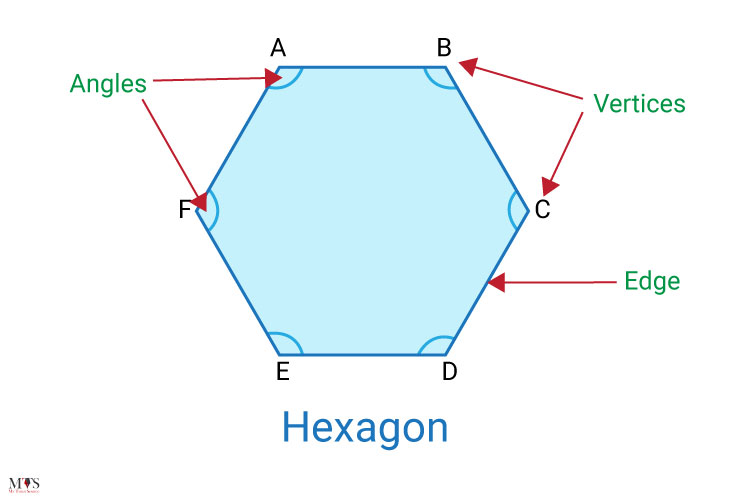# Hexagon – How many sides does a Hexagon have?## Hexagon

The two-dimensional shaped Hexagon is used in geometry. We find Hexagon shaped things everywhere, around us. Its name explains its sides quite clearly, such as:

The root word ‘Hex’ means six, a Greek prefix.

‘Gonia’ means corners.

Collectively it means six corners. Isn’t it easy to remember this 2D shape with six angles, six sides, six straight lines, six angles? To learn more about this closed 2D shape and discover what differentiates it from other polygons, give this blog post a read. We have shared the most important information about Hexagons here.

## Definition of Hexagon

A closed shape with six sides, six equal lengths, six interior angles, and six vertices is known as Hexagon. The sum of its total internal angles is 720°.

According to math tutors and professors, the most asked question during geometry class is how many sides a hexagon has or do all hexagons have six equal sides. Let us delve into the depth of hexagons and figure out how many sides all types have.

## Types of Hexagon

Hexagon shape are for following types:

• Regular hexagon
• Irregular hexagon
• Convex hexagons
• Concave hexagons

### Regular Hexagon

The properties of a regular hexagon are

• It has equal sides and angles
• Six rotational symmetries
• Six reflectional symmetries
• Interior angles are of 120° each
• Exterior angles are 60° each, equal to 360°
• The sum of its interior angles is equal to 720°
• It can be split into the same size: six equilateral triangles.

### Irregular Hexagon

The properties of an irregular hexagon are

• Six-sided two-dimensional shape
• Measurements of sides and angles are different
• Irregular in shape and size
• Internal angles are not equal to 120°. But their sum is equal to 720°

### Convex Hexagon

A convex hexagon has

• Interior angles are less than 180°
• Unequal length and sides of an angle
• No symmetric lines
• No inward pointed angles
• Vertices are pointed outwards
• Looks like the regular hexagon

### Concave Hexagon

A concave hexagon has

• Angles greater than 180°
• Only one line of symmetry across its middle
• All angles or an angle point inward

### Shapes of Hexagon

The shape of hexagon comes in two forms:

1. Regular (equal angles and side lengths)
2. Irregular (unequal angles and side lengths)

Most of the things around us are in a hexagon shape; the following are a few real-life examples:

• Pencil cross-section
• Clock
• Saturn (the planet)
• Floor tile (hexagonal)
• Honeycombs
• Patterns on footballs
• Crystals
• Beehives
• Nuts (mechanical tools)

### Sides of Hexagon

All hexagons have six sides. The sum of all sides is the perimeter of the hexagon. The sides of the hexagon have straight edges and form a closed shape.

Each side of a regular hexagon is equal in measure; however, the two sides of an irregular hexagon are different. That’s why calculating the length of regular hexagon sides is quite easier than of irregular hexagons. For example, to calculate the length of each side, divide its perimeter by 6. i.e., Perimeter ÷ 6

### Angles of Hexagon

Following are the properties related to angles of the hexagon;

• Hexagon has six exterior and six interior angles.
• The sum of all hexagon angles is 720°.
• The sum of exterior angles is 360°.
• All Irregular hexagon two angles are different in measurements.
• The measurement of each interior angle of a regular hexagon is 120°
• The measurement of each exterior angle of a regular hexagon is 60°

### Properties of Hexagon

The important properties of the hexagon are given below:

• It’s in a flat two-dimensional shape.
• It has six edges, six vertices, and six sides.
• Each side length is equal or unequal in measurement. Not all hexagons have equal angles or sides.
• The sum of its internal angles is equal to 720°
• The sum of its external angles add up to 360°
• All internal and external angles of a regular hexagon are equal to 120° and 60°, respectively.
• Hexagons have two diagonals: long diagonal and short diagonal.
• A regular hexagon is similar to a convex hexagon. their internal angles are less than 180°
• Regular hexagon opposite side is always parallel to each other.
• Add the lengths of all sides of a hexagon to find its perimeter.
• A hexagon can easily split into an equilateral triangle (six).
• It has nine diagonals.
• Hexagons with unequal length sides are known as arbitrary hexagons.

### What is the diagonal of the hexagon?

A line segment that joins two non-adjacent vertices of a polygon is called the diagonal of a hexagon. Each vertex of the hexagon has three diagonals, so, collectively, a hexagon has 18 diagonals.
Basically, the hexagon has two types of diagonals:

1. Long diagonal
2. Short diagonal

The length of each short and long diagonal can be measured using the formula √3s and 2s, respectively. Here, s represents the length of each side.

### Practice Question

#### Find out the length of each side of a regular hexagon with a perimeter of 108 units?

Solution

the perimeter of the hexagon = 108 units.
Divide the perimeter by 6.
Perimeter ÷ 6 ⇒ 108 ÷ 6
= 18 units

Hence, 18 units is the length of each hexagon’s sides.

Hexagon: a six-sided regular polygon or 6-gon

### Find Top Tutors in Your AreaWith over 3 years of experience in teaching, Chloe is very deeply connected with the topics that talk about the educational and general aspects of a student's life. Her writing has been very helpful for students to gain a better understanding of their academics and personal well-being. I’m also open to any suggestions that you might have! Please reach out to me at chloedaniel402 [at] gmail.com